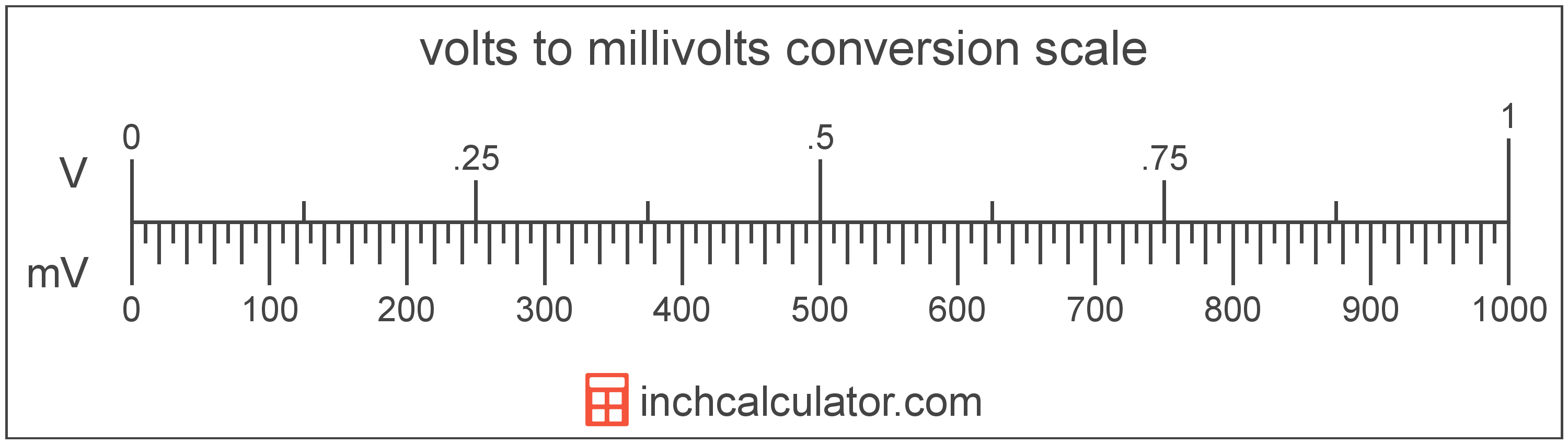# Volts to Millivolts Converter

Enter the voltage in volts below to get the value converted to millivolts.

Results in Millivolts:1 V = 1,000 mV

Do you want to convert millivolts to volts?

## How to Convert Volts to Millivolts

To convert a measurement in volts to a measurement in millivolts, multiply the voltage by the following conversion ratio: 1,000 millivolts/volt.

Since one volt is equal to 1,000 millivolts, you can use this simple formula to convert:

millivolts = volts × 1,000

The voltage in millivolts is equal to the voltage in volts multiplied by 1,000.

For example, here's how to convert 5 volts to millivolts using the formula above.
millivolts = (5 V × 1,000) = 5,000 mV### How Many Millivolts Are in a Volt?

There are 1,000 millivolts in a volt, which is why we use this value in the formula above.

1 V = 1,000 mV

## What Is a Volt?

One volt is equal to the potential difference that would move one ampere of current against one ohm of resistance.

The volt is the SI derived unit for voltage in the metric system. Volts can be abbreviated as V; for example, 1 volt can be written as 1 V.

### Voltage and Ohm's Law

Voltage is a measurement of electromotive force and the electrical potential difference between two points of a conductor.

Ohm's Law states the current between two points on a conductor is proportional to the voltage and inversely proportional to the resistance. Using Ohm's Law, it's possible to express the potential difference in volts as an expression using current and resistance.

VV = IA × R

The potential difference in volts is equal to the current in amperes times the resistance in ohms.

## What Is a Millivolt?

One millivolt is equal to 1/1,000 of a volt, which is the potential difference that would move one ampere of current against one ohm of resistance.

The millivolt is a multiple of the volt, which is the SI derived unit for voltage. In the metric system, "milli" is the prefix for thousandths, or 10-3. Millivolts can be abbreviated as mV; for example, 1 millivolt can be written as 1 mV.

## Volt to Millivolt Conversion Table

Table showing various volt measurements converted to millivolts.
Volts Millivolts
0.001 V 1 mV
0.002 V 2 mV
0.003 V 3 mV
0.004 V 4 mV
0.005 V 5 mV
0.006 V 6 mV
0.007 V 7 mV
0.008 V 8 mV
0.009 V 9 mV
0.01 V 10 mV
0.02 V 20 mV
0.03 V 30 mV
0.04 V 40 mV
0.05 V 50 mV
0.06 V 60 mV
0.07 V 70 mV
0.08 V 80 mV
0.09 V 90 mV
0.1 V 100 mV
0.2 V 200 mV
0.3 V 300 mV
0.4 V 400 mV
0.5 V 500 mV
0.6 V 600 mV
0.7 V 700 mV
0.8 V 800 mV
0.9 V 900 mV
1 V 1,000 mV

## References

1. International Bureau of Weights and Measures, The International System of Units, 9th Edition, 2019, https://www.bipm.org/documents/20126/41483022/SI-Brochure-9-EN.pdf